# Module: restoration¶

Image restoration module.

 skimage.restoration.ball_kernel(radius, ndim) Create a ball kernel for restoration.rolling_ball. Calibrate a denoising function and return optimal J-invariant version. skimage.restoration.cycle_spin(x, func, …) Cycle spinning (repeatedly apply func to shifted versions of x). Denoise image using bilateral filter. Perform non-local means denoising on 2D-4D grayscale or RGB images. Perform total-variation denoising using split-Bregman optimization. Perform total-variation denoising on n-dimensional images. skimage.restoration.denoise_wavelet(image[, …]) Perform wavelet denoising on an image. Create an ellipoid kernel for restoration.rolling_ball. skimage.restoration.estimate_sigma(image[, …]) Robust wavelet-based estimator of the (Gaussian) noise standard deviation. Inpaint masked points in image with biharmonic equations. skimage.restoration.richardson_lucy(image, psf) Richardson-Lucy deconvolution. skimage.restoration.rolling_ball(image, *[, …]) Estimate background intensity by rolling/translating a kernel. Unsupervised Wiener-Hunt deconvolution. skimage.restoration.unwrap_phase(image[, …]) Recover the original from a wrapped phase image. skimage.restoration.wiener(image, psf, balance) Wiener-Hunt deconvolution

## ball_kernel¶

skimage.restoration.ball_kernel(radius, ndim)[source]

Create a ball kernel for restoration.rolling_ball.

Parameters

ndimint

Number of dimensions of the ball. ndim should match the dimensionality of the image the kernel will be applied to.

Returns
kernelndarray

The kernel containing the surface intensity of the top half of the ellipsoid.

## calibrate_denoiser¶

skimage.restoration.calibrate_denoiser(image, denoise_function, denoise_parameters, *, stride=4, approximate_loss=True, extra_output=False)[source]

Calibrate a denoising function and return optimal J-invariant version.

The returned function is partially evaluated with optimal parameter values set for denoising the input image.

Parameters
imagendarray

Input data to be denoised (converted using img_as_float).

denoise_functionfunction

Denoising function to be calibrated.

denoise_parametersdict of list

Ranges of parameters for denoise_function to be calibrated over.

strideint, optional

Stride used in masking procedure that converts denoise_function to J-invariance.

approximate_lossbool, optional

Whether to approximate the self-supervised loss used to evaluate the denoiser by only computing it on one masked version of the image. If False, the runtime will be a factor of stride**image.ndim longer.

extra_outputbool, optional

If True, return parameters and losses in addition to the calibrated denoising function

Returns
best_denoise_functionfunction

The optimal J-invariant version of denoise_function.

If extra_output is True, the following tuple is also returned:
(parameters_tested, losses)tuple (list of dict, list of int)

List of parameters tested for denoise_function, as a dictionary of kwargs Self-supervised loss for each set of parameters in parameters_tested.

Notes

The calibration procedure uses a self-supervised mean-square-error loss to evaluate the performance of J-invariant versions of denoise_function. The minimizer of the self-supervised loss is also the minimizer of the ground-truth loss (i.e., the true MSE error) . The returned function can be used on the original noisy image, or other images with similar characteristics.

Increasing the stride increases the performance of best_denoise_function

at the expense of increasing its runtime. It has no effect on the runtime of the calibration.

References

1

J. Batson & L. Royer. Noise2Self: Blind Denoising by Self-Supervision, International Conference on Machine Learning, p. 524-533 (2019).

Examples

>>> from skimage import color, data
>>> from skimage.restoration import denoise_wavelet
>>> import numpy as np
>>> img = color.rgb2gray(data.astronaut()[:50, :50])
>>> rng = np.random.default_rng()
>>> noisy = img + 0.5 * img.std() * rng.standard_normal(img.shape)
>>> parameters = {'sigma': np.arange(0.1, 0.4, 0.02)}
>>> denoising_function = calibrate_denoiser(noisy, denoise_wavelet,
...                                         denoise_parameters=parameters)
>>> denoised_img = denoising_function(img)


### Examples using skimage.restoration.calibrate_denoiser¶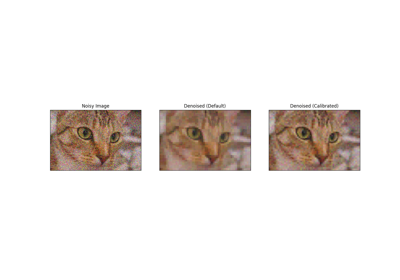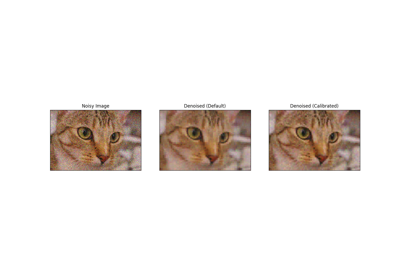## cycle_spin¶

skimage.restoration.cycle_spin(x, func, max_shifts, shift_steps=1, num_workers=None, multichannel=False, func_kw={}, *, channel_axis=- 1)[source]

Cycle spinning (repeatedly apply func to shifted versions of x).

Parameters
xarray-like

Data for input to func.

funcfunction

A function to apply to circularly shifted versions of x. Should take x as its first argument. Any additional arguments can be supplied via func_kw.

max_shiftsint or tuple

If an integer, shifts in range(0, max_shifts+1) will be used along each axis of x. If a tuple, range(0, max_shifts[i]+1) will be along axis i.

shift_stepsint or tuple, optional

The step size for the shifts applied along axis, i, are:: range((0, max_shifts[i]+1, shift_steps[i])). If an integer is provided, the same step size is used for all axes.

num_workersint or None, optional

The number of parallel threads to use during cycle spinning. If set to None, the full set of available cores are used.

multichannelbool, optional

Whether to treat the final axis as channels (no cycle shifts are performed over the channels axis). This argument is deprecated: specify channel_axis instead.

func_kwdict, optional

Additional keyword arguments to supply to func.

channel_axisint or None, optional

If None, the image is assumed to be a grayscale (single channel) image. Otherwise, this parameter indicates which axis of the array corresponds to channels.

New in version 0.19: channel_axis was added in 0.19.

Returns
avg_ynp.ndarray

The output of func(x, **func_kw) averaged over all combinations of the specified axis shifts.

Other Parameters
multichannelDEPRECATED

Deprecated in favor of channel_axis.

Deprecated since version 0.19.

Notes

Cycle spinning was proposed as a way to approach shift-invariance via performing several circular shifts of a shift-variant transform .

For a n-level discrete wavelet transforms, one may wish to perform all shifts up to max_shifts = 2**n - 1. In practice, much of the benefit can often be realized with only a small number of shifts per axis.

For transforms such as the blockwise discrete cosine transform, one may wish to evaluate shifts up to the block size used by the transform.

References

1

R.R. Coifman and D.L. Donoho. “Translation-Invariant De-Noising”. Wavelets and Statistics, Lecture Notes in Statistics, vol.103. Springer, New York, 1995, pp.125-150. DOI:10.1007/978-1-4612-2544-7_9

Examples

>>> import skimage.data
>>> from skimage import img_as_float
>>> from skimage.restoration import denoise_wavelet, cycle_spin
>>> img = img_as_float(skimage.data.camera())
>>> sigma = 0.1
>>> img = img + sigma * np.random.standard_normal(img.shape)
>>> denoised = cycle_spin(img, func=denoise_wavelet,
...                       max_shifts=3)


### Examples using skimage.restoration.cycle_spin¶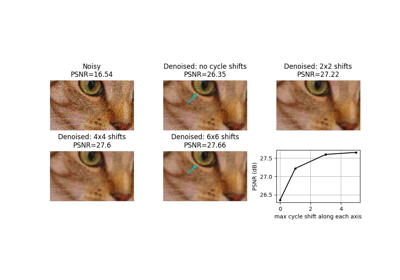## denoise_bilateral¶

skimage.restoration.denoise_bilateral(image, win_size=None, sigma_color=None, sigma_spatial=1, bins=10000, mode='constant', cval=0, multichannel=False, *, channel_axis=None)[source]

Denoise image using bilateral filter.

Parameters
imagendarray, shape (M, N[, 3])

Input image, 2D grayscale or RGB.

win_sizeint

Window size for filtering. If win_size is not specified, it is calculated as max(5, 2 * ceil(3 * sigma_spatial) + 1).

sigma_colorfloat

Standard deviation for grayvalue/color distance (radiometric similarity). A larger value results in averaging of pixels with larger radiometric differences. If None, the standard deviation of image will be used.

sigma_spatialfloat

Standard deviation for range distance. A larger value results in averaging of pixels with larger spatial differences.

binsint

Number of discrete values for Gaussian weights of color filtering. A larger value results in improved accuracy.

mode{‘constant’, ‘edge’, ‘symmetric’, ‘reflect’, ‘wrap’}

How to handle values outside the image borders. See numpy.pad for detail.

cvalstring

Used in conjunction with mode ‘constant’, the value outside the image boundaries.

multichannelbool

Whether the last axis of the image is to be interpreted as multiple channels or another spatial dimension. This argument is deprecated: specify channel_axis instead.

channel_axisint or None, optional

If None, the image is assumed to be a grayscale (single channel) image. Otherwise, this parameter indicates which axis of the array corresponds to channels.

New in version 0.19: channel_axis was added in 0.19.

Returns
denoisedndarray

Denoised image.

Other Parameters
multichannelDEPRECATED

Deprecated in favor of channel_axis.

Deprecated since version 0.19.

Notes

This is an edge-preserving, denoising filter. It averages pixels based on their spatial closeness and radiometric similarity .

Spatial closeness is measured by the Gaussian function of the Euclidean distance between two pixels and a certain standard deviation (sigma_spatial).

Radiometric similarity is measured by the Gaussian function of the Euclidean distance between two color values and a certain standard deviation (sigma_color).

Note that, if the image is of any int dtype, image will be converted using the img_as_float function and thus the standard deviation (sigma_color) will be in range [0, 1].

For more information on scikit-image’s data type conversions and how images are rescaled in these conversions, see: https://scikit-image.org/docs/stable/user_guide/data_types.html.

References

1

C. Tomasi and R. Manduchi. “Bilateral Filtering for Gray and Color Images.” IEEE International Conference on Computer Vision (1998) 839-846. DOI:10.1109/ICCV.1998.710815

Examples

>>> from skimage import data, img_as_float
>>> astro = img_as_float(data.astronaut())
>>> astro = astro[220:300, 220:320]
>>> rng = np.random.default_rng()
>>> noisy = astro + 0.6 * astro.std() * rng.random(astro.shape)
>>> noisy = np.clip(noisy, 0, 1)
>>> denoised = denoise_bilateral(noisy, sigma_color=0.05, sigma_spatial=15,
...                              channel_axis=-1)


### Examples using skimage.restoration.denoise_bilateral¶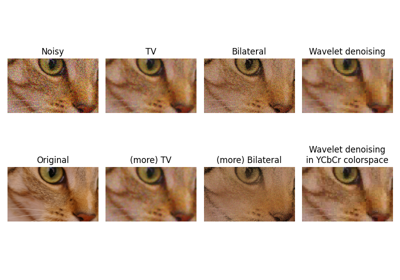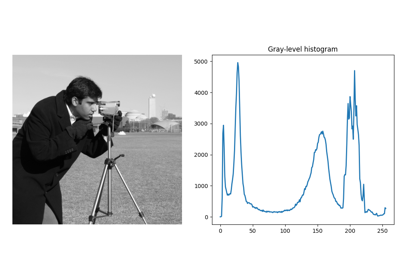## denoise_nl_means¶

skimage.restoration.denoise_nl_means(image, patch_size=7, patch_distance=11, h=0.1, multichannel=False, fast_mode=True, sigma=0.0, *, preserve_range=False, channel_axis=None)[source]

Perform non-local means denoising on 2D-4D grayscale or RGB images.

Parameters
image2D or 3D ndarray

Input image to be denoised, which can be 2D or 3D, and grayscale or RGB (for 2D images only, see multichannel parameter). There can be any number of channels (does not strictly have to be RGB).

patch_sizeint, optional

Size of patches used for denoising.

patch_distanceint, optional

Maximal distance in pixels where to search patches used for denoising.

hfloat, optional

Cut-off distance (in gray levels). The higher h, the more permissive one is in accepting patches. A higher h results in a smoother image, at the expense of blurring features. For a Gaussian noise of standard deviation sigma, a rule of thumb is to choose the value of h to be sigma of slightly less.

multichannelbool, optional

Whether the last axis of the image is to be interpreted as multiple channels or another spatial dimension. This argument is deprecated: specify channel_axis instead.

fast_modebool, optional

If True (default value), a fast version of the non-local means algorithm is used. If False, the original version of non-local means is used. See the Notes section for more details about the algorithms.

sigmafloat, optional

The standard deviation of the (Gaussian) noise. If provided, a more robust computation of patch weights is computed that takes the expected noise variance into account (see Notes below).

preserve_rangebool, optional

Whether to keep the original range of values. Otherwise, the input image is converted according to the conventions of img_as_float. Also see https://scikit-image.org/docs/dev/user_guide/data_types.html

channel_axisint or None, optional

If None, the image is assumed to be a grayscale (single channel) image. Otherwise, this parameter indicates which axis of the array corresponds to channels.

New in version 0.19: channel_axis was added in 0.19.

Returns
resultndarray

Denoised image, of same shape as image.

Other Parameters
multichannelDEPRECATED

Deprecated in favor of channel_axis.

Deprecated since version 0.19.

Notes

The non-local means algorithm is well suited for denoising images with specific textures. The principle of the algorithm is to average the value of a given pixel with values of other pixels in a limited neighbourhood, provided that the patches centered on the other pixels are similar enough to the patch centered on the pixel of interest.

In the original version of the algorithm , corresponding to fast=False, the computational complexity is:

image.size * patch_size ** image.ndim * patch_distance ** image.ndim


Hence, changing the size of patches or their maximal distance has a strong effect on computing times, especially for 3-D images.

However, the default behavior corresponds to fast_mode=True, for which another version of non-local means  is used, corresponding to a complexity of:

image.size * patch_distance ** image.ndim


The computing time depends only weakly on the patch size, thanks to the computation of the integral of patches distances for a given shift, that reduces the number of operations . Therefore, this algorithm executes faster than the classic algorithm (fast_mode=False), at the expense of using twice as much memory. This implementation has been proven to be more efficient compared to other alternatives, see e.g. .

Compared to the classic algorithm, all pixels of a patch contribute to the distance to another patch with the same weight, no matter their distance to the center of the patch. This coarser computation of the distance can result in a slightly poorer denoising performance. Moreover, for small images (images with a linear size that is only a few times the patch size), the classic algorithm can be faster due to boundary effects.

The image is padded using the reflect mode of skimage.util.pad before denoising.

If the noise standard deviation, sigma, is provided a more robust computation of patch weights is used. Subtracting the known noise variance from the computed patch distances improves the estimates of patch similarity, giving a moderate improvement to denoising performance . It was also mentioned as an option for the fast variant of the algorithm in .

When sigma is provided, a smaller h should typically be used to avoid oversmoothing. The optimal value for h depends on the image content and noise level, but a reasonable starting point is h = 0.8 * sigma when fast_mode is True, or h = 0.6 * sigma when fast_mode is False.

References

1(1,2)

A. Buades, B. Coll, & J-M. Morel. A non-local algorithm for image denoising. In CVPR 2005, Vol. 2, pp. 60-65, IEEE. DOI:10.1109/CVPR.2005.38

2

J. Darbon, A. Cunha, T.F. Chan, S. Osher, and G.J. Jensen, Fast nonlocal filtering applied to electron cryomicroscopy, in 5th IEEE International Symposium on Biomedical Imaging: From Nano to Macro, 2008, pp. 1331-1334. DOI:10.1109/ISBI.2008.4541250

3(1,2)

Jacques Froment. Parameter-Free Fast Pixelwise Non-Local Means Denoising. Image Processing On Line, 2014, vol. 4, pp. 300-326. DOI:10.5201/ipol.2014.120

4

A. Buades, B. Coll, & J-M. Morel. Non-Local Means Denoising. Image Processing On Line, 2011, vol. 1, pp. 208-212. DOI:10.5201/ipol.2011.bcm_nlm

Examples

>>> a = np.zeros((40, 40))
>>> a[10:-10, 10:-10] = 1.
>>> rng = np.random.default_rng()
>>> a += 0.3 * rng.standard_normal(a.shape)
>>> denoised_a = denoise_nl_means(a, 7, 5, 0.1)


### Examples using skimage.restoration.denoise_nl_means¶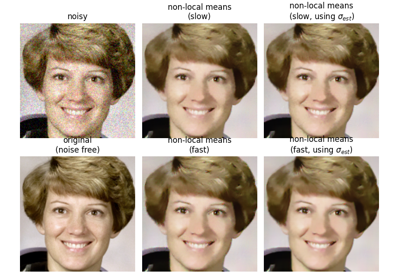## denoise_tv_bregman¶

skimage.restoration.denoise_tv_bregman(image, weight=5.0, max_num_iter=100, eps=0.001, isotropic=True, *, channel_axis=None, multichannel=False)[source]

Perform total-variation denoising using split-Bregman optimization.

Total-variation denoising (also know as total-variation regularization) tries to find an image with less total-variation under the constraint of being similar to the input image, which is controlled by the regularization parameter (, , , ).

Parameters
imagendarray

Input data to be denoised (converted using img_as_float).

weightfloat

Denoising weight. The smaller the weight, the more denoising (at the expense of less similarity to the input). The regularization parameter lambda is chosen as 2 * weight.

epsfloat, optional

Relative difference of the value of the cost function that determines the stop criterion. The algorithm stops when:

SUM((u(n) - u(n-1))**2) < eps

max_num_iterint, optional

Maximal number of iterations used for the optimization.

isotropicboolean, optional

Switch between isotropic and anisotropic TV denoising.

channel_axisint or None, optional

If None, the image is assumed to be a grayscale (single channel) image. Otherwise, this parameter indicates which axis of the array corresponds to channels.

New in version 0.19: channel_axis was added in 0.19.

multichannelbool, optional

Apply total-variation denoising separately for each channel. This option should be true for color images, otherwise the denoising is also applied in the channels dimension. This argument is deprecated: specify channel_axis instead.

Returns
undarray

Denoised image.

Other Parameters
max_iterDEPRECATED

Deprecated in favor of max_num_iter.

Deprecated since version 0.19.

multichannelDEPRECATED

Deprecated in favor of channel_axis.

Deprecated since version 0.19.

References

1

https://en.wikipedia.org/wiki/Total_variation_denoising

2

Tom Goldstein and Stanley Osher, “The Split Bregman Method For L1 Regularized Problems”, ftp://ftp.math.ucla.edu/pub/camreport/cam08-29.pdf

3

Pascal Getreuer, “Rudin–Osher–Fatemi Total Variation Denoising using Split Bregman” in Image Processing On Line on 2012–05–19, https://www.ipol.im/pub/art/2012/g-tvd/article_lr.pdf

4

https://web.math.ucsb.edu/~cgarcia/UGProjects/BregmanAlgorithms_JacquelineBush.pdf

## denoise_tv_chambolle¶

skimage.restoration.denoise_tv_chambolle(image, weight=0.1, eps=0.0002, max_num_iter=200, multichannel=False, *, channel_axis=None)[source]

Perform total-variation denoising on n-dimensional images.

Parameters
imagendarray of ints, uints or floats

Input data to be denoised. image can be of any numeric type, but it is cast into an ndarray of floats for the computation of the denoised image.

weightfloat, optional

Denoising weight. The greater weight, the more denoising (at the expense of fidelity to input).

epsfloat, optional

Relative difference of the value of the cost function that determines the stop criterion. The algorithm stops when:

(E_(n-1) - E_n) < eps * E_0

max_num_iterint, optional

Maximal number of iterations used for the optimization.

multichannelbool, optional

Apply total-variation denoising separately for each channel. This option should be true for color images, otherwise the denoising is also applied in the channels dimension. This argument is deprecated: specify channel_axis instead.

channel_axisint or None, optional

If None, the image is assumed to be a grayscale (single channel) image. Otherwise, this parameter indicates which axis of the array corresponds to channels.

New in version 0.19: channel_axis was added in 0.19.

Returns
outndarray

Denoised image.

Other Parameters
multichannelDEPRECATED

Deprecated in favor of channel_axis.

Deprecated since version 0.19.

n_iter_maxDEPRECATED

Deprecated in favor of max_num_iter.

Deprecated since version 0.19.2.

Notes

Make sure to set the multichannel parameter appropriately for color images.

The principle of total variation denoising is explained in https://en.wikipedia.org/wiki/Total_variation_denoising

The principle of total variation denoising is to minimize the total variation of the image, which can be roughly described as the integral of the norm of the image gradient. Total variation denoising tends to produce “cartoon-like” images, that is, piecewise-constant images.

This code is an implementation of the algorithm of Rudin, Fatemi and Osher that was proposed by Chambolle in .

References

1

A. Chambolle, An algorithm for total variation minimization and applications, Journal of Mathematical Imaging and Vision, Springer, 2004, 20, 89-97.

Examples

2D example on astronaut image:

>>> from skimage import color, data
>>> img = color.rgb2gray(data.astronaut())[:50, :50]
>>> rng = np.random.default_rng()
>>> img += 0.5 * img.std() * rng.standard_normal(img.shape)
>>> denoised_img = denoise_tv_chambolle(img, weight=60)


3D example on synthetic data:

>>> x, y, z = np.ogrid[0:20, 0:20, 0:20]
>>> mask = (x - 22)**2 + (y - 20)**2 + (z - 17)**2 < 8**2
>>> rng = np.random.default_rng()


### Examples using skimage.restoration.denoise_tv_chambolle¶## denoise_wavelet¶

skimage.restoration.denoise_wavelet(image, sigma=None, wavelet='db1', mode='soft', wavelet_levels=None, multichannel=False, convert2ycbcr=False, method='BayesShrink', rescale_sigma=True, *, channel_axis=None)[source]

Perform wavelet denoising on an image.

Parameters
imagendarray ([M[, N[, …P]][, C]) of ints, uints or floats

Input data to be denoised. image can be of any numeric type, but it is cast into an ndarray of floats for the computation of the denoised image.

sigmafloat or list, optional

The noise standard deviation used when computing the wavelet detail coefficient threshold(s). When None (default), the noise standard deviation is estimated via the method in .

waveletstring, optional

The type of wavelet to perform and can be any of the options pywt.wavelist outputs. The default is ‘db1’. For example, wavelet can be any of {'db2', 'haar', 'sym9'} and many more.

mode{‘soft’, ‘hard’}, optional

An optional argument to choose the type of denoising performed. It noted that choosing soft thresholding given additive noise finds the best approximation of the original image.

wavelet_levelsint or None, optional

The number of wavelet decomposition levels to use. The default is three less than the maximum number of possible decomposition levels.

multichannelbool, optional

Apply wavelet denoising separately for each channel (where channels correspond to the final axis of the array). This argument is deprecated: specify channel_axis instead.

convert2ycbcrbool, optional

If True and multichannel True, do the wavelet denoising in the YCbCr colorspace instead of the RGB color space. This typically results in better performance for RGB images.

method{‘BayesShrink’, ‘VisuShrink’}, optional

Thresholding method to be used. The currently supported methods are “BayesShrink”  and “VisuShrink” . Defaults to “BayesShrink”.

rescale_sigmabool, optional

If False, no rescaling of the user-provided sigma will be performed. The default of True rescales sigma appropriately if the image is rescaled internally.

New in version 0.16: rescale_sigma was introduced in 0.16

channel_axisint or None, optional

If None, the image is assumed to be a grayscale (single channel) image. Otherwise, this parameter indicates which axis of the array corresponds to channels.

New in version 0.19: channel_axis was added in 0.19.

Returns
outndarray

Denoised image.

Other Parameters
multichannelDEPRECATED

Deprecated in favor of channel_axis.

Deprecated since version 0.19.

Notes

The wavelet domain is a sparse representation of the image, and can be thought of similarly to the frequency domain of the Fourier transform. Sparse representations have most values zero or near-zero and truly random noise is (usually) represented by many small values in the wavelet domain. Setting all values below some threshold to 0 reduces the noise in the image, but larger thresholds also decrease the detail present in the image.

If the input is 3D, this function performs wavelet denoising on each color plane separately.

Changed in version 0.16: For floating point inputs, the original input range is maintained and there is no clipping applied to the output. Other input types will be converted to a floating point value in the range [-1, 1] or [0, 1] depending on the input image range. Unless rescale_sigma = False, any internal rescaling applied to the image will also be applied to sigma to maintain the same relative amplitude.

Many wavelet coefficient thresholding approaches have been proposed. By default, denoise_wavelet applies BayesShrink, which is an adaptive thresholding method that computes separate thresholds for each wavelet sub-band as described in .

If method == "VisuShrink", a single “universal threshold” is applied to all wavelet detail coefficients as described in . This threshold is designed to remove all Gaussian noise at a given sigma with high probability, but tends to produce images that appear overly smooth.

Although any of the wavelets from PyWavelets can be selected, the thresholding methods assume an orthogonal wavelet transform and may not choose the threshold appropriately for biorthogonal wavelets. Orthogonal wavelets are desirable because white noise in the input remains white noise in the subbands. Biorthogonal wavelets lead to colored noise in the subbands. Additionally, the orthogonal wavelets in PyWavelets are orthonormal so that noise variance in the subbands remains identical to the noise variance of the input. Example orthogonal wavelets are the Daubechies (e.g. ‘db2’) or symmlet (e.g. ‘sym2’) families.

References

1(1,2)

Chang, S. Grace, Bin Yu, and Martin Vetterli. “Adaptive wavelet thresholding for image denoising and compression.” Image Processing, IEEE Transactions on 9.9 (2000): 1532-1546. DOI:10.1109/83.862633

2(1,2,3)

D. L. Donoho and I. M. Johnstone. “Ideal spatial adaptation by wavelet shrinkage.” Biometrika 81.3 (1994): 425-455. DOI:10.1093/biomet/81.3.425

Examples

>>> from skimage import color, data
>>> img = img_as_float(data.astronaut())
>>> img = color.rgb2gray(img)
>>> rng = np.random.default_rng()
>>> img += 0.1 * rng.standard_normal(img.shape)
>>> img = np.clip(img, 0, 1)
>>> denoised_img = denoise_wavelet(img, sigma=0.1, rescale_sigma=True)


### Examples using skimage.restoration.denoise_wavelet¶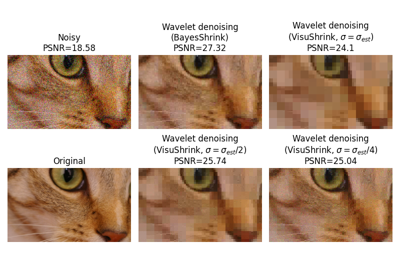## ellipsoid_kernel¶

skimage.restoration.ellipsoid_kernel(shape, intensity)[source]

Create an ellipoid kernel for restoration.rolling_ball.

Parameters
shapearraylike

Length of the principal axis of the ellipsoid (excluding the intensity axis). The kernel needs to have the same dimensionality as the image it will be applied to.

intensityint

Length of the intensity axis of the ellipsoid.

Returns
kernelndarray

The kernel containing the surface intensity of the top half of the ellipsoid.

### Examples using skimage.restoration.ellipsoid_kernel¶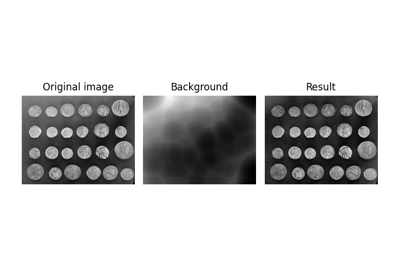## estimate_sigma¶

skimage.restoration.estimate_sigma(image, average_sigmas=False, multichannel=False, *, channel_axis=None)[source]

Robust wavelet-based estimator of the (Gaussian) noise standard deviation.

Parameters
imagendarray

Image for which to estimate the noise standard deviation.

average_sigmasbool, optional

If true, average the channel estimates of sigma. Otherwise return a list of sigmas corresponding to each channel.

multichannelbool

Estimate sigma separately for each channel. This argument is deprecated: specify channel_axis instead.

channel_axisint or None, optional

If None, the image is assumed to be a grayscale (single channel) image. Otherwise, this parameter indicates which axis of the array corresponds to channels.

New in version 0.19: channel_axis was added in 0.19.

Returns
sigmafloat or list

Estimated noise standard deviation(s). If multichannel is True and average_sigmas is False, a separate noise estimate for each channel is returned. Otherwise, the average of the individual channel estimates is returned.

Other Parameters
multichannelDEPRECATED

Deprecated in favor of channel_axis.

Deprecated since version 0.19.

Notes

This function assumes the noise follows a Gaussian distribution. The estimation algorithm is based on the median absolute deviation of the wavelet detail coefficients as described in section 4.2 of .

References

1

D. L. Donoho and I. M. Johnstone. “Ideal spatial adaptation by wavelet shrinkage.” Biometrika 81.3 (1994): 425-455. DOI:10.1093/biomet/81.3.425

Examples

>>> import skimage.data
>>> from skimage import img_as_float
>>> img = img_as_float(skimage.data.camera())
>>> sigma = 0.1
>>> rng = np.random.default_rng()
>>> img = img + sigma * rng.standard_normal(img.shape)
>>> sigma_hat = estimate_sigma(img, channel_axis=None)


### Examples using skimage.restoration.estimate_sigma¶## inpaint_biharmonic¶

skimage.restoration.inpaint_biharmonic(image, mask, multichannel=False, *, split_into_regions=False, channel_axis=None)[source]

Inpaint masked points in image with biharmonic equations.

Parameters
image(M[, N[, …, P]][, C]) ndarray

Input image.

Array of pixels to be inpainted. Have to be the same shape as one of the ‘image’ channels. Unknown pixels have to be represented with 1, known pixels - with 0.

multichannelboolean, optional

If True, the last image dimension is considered as a color channel, otherwise as spatial. This argument is deprecated: specify channel_axis instead.

split_into_regionsboolean, optional

If True, inpainting is performed on a region-by-region basis. This is likely to be slower, but will have reduced memory requirements.

channel_axisint or None, optional

If None, the image is assumed to be a grayscale (single channel) image. Otherwise, this parameter indicates which axis of the array corresponds to channels.

New in version 0.19: channel_axis was added in 0.19.

Returns
out(M[, N[, …, P]][, C]) ndarray

Input image with masked pixels inpainted.

Other Parameters
multichannelDEPRECATED

Deprecated in favor of channel_axis.

Deprecated since version 0.19.

References

1

S.B.Damelin and N.S.Hoang. “On Surface Completion and Image Inpainting by Biharmonic Functions: Numerical Aspects”, International Journal of Mathematics and Mathematical Sciences, Vol. 2018, Article ID 3950312 DOI:10.1155/2018/3950312

2

C. K. Chui and H. N. Mhaskar, MRA Contextual-Recovery Extension of Smooth Functions on Manifolds, Appl. and Comp. Harmonic Anal., 28 (2010), 104-113, DOI:10.1016/j.acha.2009.04.004

Examples

>>> img = np.tile(np.square(np.linspace(0, 1, 5)), (5, 1))


### Examples using skimage.restoration.inpaint_biharmonic¶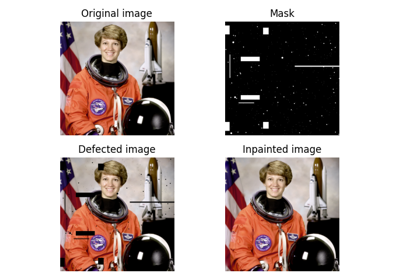## richardson_lucy¶

skimage.restoration.richardson_lucy(image, psf, num_iter=50, clip=True, filter_epsilon=None)[source]

Richardson-Lucy deconvolution.

Parameters
imagendarray

Input degraded image (can be N dimensional).

psfndarray

num_iterint, optional

Number of iterations. This parameter plays the role of regularisation.

clipboolean, optional

True by default. If true, pixel value of the result above 1 or under -1 are thresholded for skimage pipeline compatibility.

filter_epsilon: float, optional

Value below which intermediate results become 0 to avoid division by small numbers.

Returns
im_deconvndarray

The deconvolved image.

Other Parameters
iterationsDEPRECATED

Deprecated in favor of num_iter.

Deprecated since version 0.19.

References

1

https://en.wikipedia.org/wiki/Richardson%E2%80%93Lucy_deconvolution

Examples

>>> from skimage import img_as_float, data, restoration
>>> camera = img_as_float(data.camera())
>>> from scipy.signal import convolve2d
>>> psf = np.ones((5, 5)) / 25
>>> camera = convolve2d(camera, psf, 'same')
>>> rng = np.random.default_rng()
>>> camera += 0.1 * camera.std() * rng.standard_normal(camera.shape)
>>> deconvolved = restoration.richardson_lucy(camera, psf, 5)


### Examples using skimage.restoration.richardson_lucy¶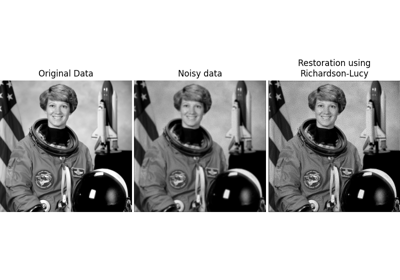## rolling_ball¶

skimage.restoration.rolling_ball(image, *, radius=100, kernel=None, nansafe=False, num_threads=None)[source]

Estimate background intensity by rolling/translating a kernel.

This rolling ball algorithm estimates background intensity for a ndimage in case of uneven exposure. It is a generalization of the frequently used rolling ball algorithm .

Parameters
imagendarray

The image to be filtered.

Radius of a ball shaped kernel to be rolled/translated in the image. Used if kernel = None.

kernelndarray, optional

The kernel to be rolled/translated in the image. It must have the same number of dimensions as image. Kernel is filled with the intensity of the kernel at that position.

nansafe: bool, optional

If False (default) assumes that none of the values in image are np.nan, and uses a faster implementation.

The maximum number of threads to use. If None use the OpenMP default value; typically equal to the maximum number of virtual cores. Note: This is an upper limit to the number of threads. The exact number is determined by the system’s OpenMP library.

Returns
backgroundndarray

The estimated background of the image.

Notes

For the pixel that has its background intensity estimated (without loss of generality at center) the rolling ball method centers kernel under it and raises the kernel until the surface touches the image umbra at some pos=(y,x). The background intensity is then estimated using the image intensity at that position (image[pos]) plus the difference of kernel[center] - kernel[pos].

This algorithm assumes that dark pixels correspond to the background. If you have a bright background, invert the image before passing it to the function, e.g., using utils.invert. See the gallery example for details.

This algorithm is sensitive to noise (in particular salt-and-pepper noise). If this is a problem in your image, you can apply mild gaussian smoothing before passing the image to this function.

References

1

Sternberg, Stanley R. “Biomedical image processing.” Computer 1 (1983): 22-34. DOI:10.1109/MC.1983.1654163

Examples

>>> import numpy as np
>>> from skimage import data
>>> from skimage.restoration import rolling_ball
>>> image = data.coins()
>>> background = rolling_ball(data.coins())
>>> filtered_image = image - background

>>> import numpy as np
>>> from skimage import data
>>> from skimage.restoration import rolling_ball, ellipsoid_kernel
>>> image = data.coins()
>>> kernel = ellipsoid_kernel((101, 101), 75)
>>> background = rolling_ball(data.coins(), kernel=kernel)
>>> filtered_image = image - background


### Examples using skimage.restoration.rolling_ball¶## unsupervised_wiener¶

skimage.restoration.unsupervised_wiener(image, psf, reg=None, user_params=None, is_real=True, clip=True, *, random_state=None)[source]

Unsupervised Wiener-Hunt deconvolution.

Return the deconvolution with a Wiener-Hunt approach, where the hyperparameters are automatically estimated. The algorithm is a stochastic iterative process (Gibbs sampler) described in the reference below. See also wiener function.

Parameters
image(M, N) ndarray

psfndarray

The impulse response (input image’s space) or the transfer function (Fourier space). Both are accepted. The transfer function is automatically recognized as being complex (np.iscomplexobj(psf)).

regndarray, optional

The regularisation operator. The Laplacian by default. It can be an impulse response or a transfer function, as for the psf.

user_paramsdict, optional

Dictionary of parameters for the Gibbs sampler. See below.

clipboolean, optional

True by default. If true, pixel values of the result above 1 or under -1 are thresholded for skimage pipeline compatibility.

random_state{None, int, numpy.random.Generator}, optional

If random_state is None the numpy.random.Generator singleton is used. If random_state is an int, a new Generator instance is used, seeded with random_state. If random_state is already a Generator instance then that instance is used.

New in version 0.19.

Returns
x_postmean(M, N) ndarray

The deconvolved image (the posterior mean).

chainsdict

The keys noise and prior contain the chain list of noise and prior precision respectively.

Other Parameters
The keys of user_params are:
thresholdfloat

The stopping criterion: the norm of the difference between to successive approximated solution (empirical mean of object samples, see Notes section). 1e-4 by default.

burninint

The number of sample to ignore to start computation of the mean. 15 by default.

min_num_iterint

The minimum number of iterations. 30 by default.

max_num_iterint

The maximum number of iterations if threshold is not satisfied. 200 by default.

callbackcallable (None by default)

A user provided callable to which is passed, if the function exists, the current image sample for whatever purpose. The user can store the sample, or compute other moments than the mean. It has no influence on the algorithm execution and is only for inspection.

Notes

The estimated image is design as the posterior mean of a probability law (from a Bayesian analysis). The mean is defined as a sum over all the possible images weighted by their respective probability. Given the size of the problem, the exact sum is not tractable. This algorithm use of MCMC to draw image under the posterior law. The practical idea is to only draw highly probable images since they have the biggest contribution to the mean. At the opposite, the less probable images are drawn less often since their contribution is low. Finally the empirical mean of these samples give us an estimation of the mean, and an exact computation with an infinite sample set.

References

1

François Orieux, Jean-François Giovannelli, and Thomas Rodet, “Bayesian estimation of regularization and point spread function parameters for Wiener-Hunt deconvolution”, J. Opt. Soc. Am. A 27, 1593-1607 (2010)

https://www.osapublishing.org/josaa/abstract.cfm?URI=josaa-27-7-1593

http://research.orieux.fr/files/papers/OGR-JOSA10.pdf

Examples

>>> from skimage import color, data, restoration
>>> img = color.rgb2gray(data.astronaut())
>>> from scipy.signal import convolve2d
>>> psf = np.ones((5, 5)) / 25
>>> img = convolve2d(img, psf, 'same')
>>> rng = np.random.default_rng()
>>> img += 0.1 * img.std() * rng.standard_normal(img.shape)
>>> deconvolved_img = restoration.unsupervised_wiener(img, psf)


### Examples using skimage.restoration.unsupervised_wiener¶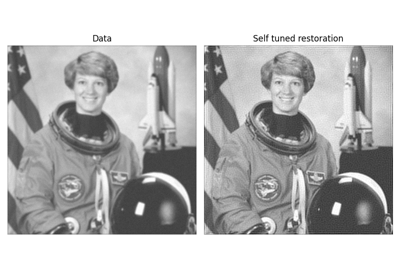## unwrap_phase¶

skimage.restoration.unwrap_phase(image, wrap_around=False, seed=None)[source]

Recover the original from a wrapped phase image.

From an image wrapped to lie in the interval [-pi, pi), recover the original, unwrapped image.

Parameters
image1D, 2D or 3D ndarray of floats, optionally a masked array

The values should be in the range [-pi, pi). If a masked array is provided, the masked entries will not be changed, and their values will not be used to guide the unwrapping of neighboring, unmasked values. Masked 1D arrays are not allowed, and will raise a ValueError.

wrap_aroundbool or sequence of bool, optional

When an element of the sequence is True, the unwrapping process will regard the edges along the corresponding axis of the image to be connected and use this connectivity to guide the phase unwrapping process. If only a single boolean is given, it will apply to all axes. Wrap around is not supported for 1D arrays.

seedint, optional

Unwrapping 2D or 3D images uses random initialization. This sets the seed of the PRNG to achieve deterministic behavior.

Returns
image_unwrappedarray_like, double

Unwrapped image of the same shape as the input. If the input image was a masked array, the mask will be preserved.

Raises
ValueError

If called with a masked 1D array or called with a 1D array and wrap_around=True.

References

1

Miguel Arevallilo Herraez, David R. Burton, Michael J. Lalor, and Munther A. Gdeisat, “Fast two-dimensional phase-unwrapping algorithm based on sorting by reliability following a noncontinuous path”, Journal Applied Optics, Vol. 41, No. 35 (2002) 7437,

2

Abdul-Rahman, H., Gdeisat, M., Burton, D., & Lalor, M., “Fast three-dimensional phase-unwrapping algorithm based on sorting by reliability following a non-continuous path. In W. Osten, C. Gorecki, & E. L. Novak (Eds.), Optical Metrology (2005) 32–40, International Society for Optics and Photonics.

Examples

>>> c0, c1 = np.ogrid[-1:1:128j, -1:1:128j]
>>> image = 12 * np.pi * np.exp(-(c0**2 + c1**2))
>>> image_wrapped = np.angle(np.exp(1j * image))
>>> image_unwrapped = unwrap_phase(image_wrapped)
>>> np.std(image_unwrapped - image) < 1e-6   # A constant offset is normal
True


### Examples using skimage.restoration.unwrap_phase¶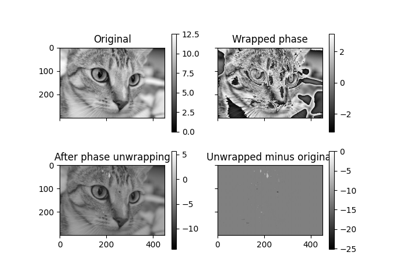## wiener¶

skimage.restoration.wiener(image, psf, balance, reg=None, is_real=True, clip=True)[source]

Wiener-Hunt deconvolution

Return the deconvolution with a Wiener-Hunt approach (i.e. with Fourier diagonalisation).

Parameters
image(M, N) ndarray

psfndarray

Point Spread Function. This is assumed to be the impulse response (input image space) if the data-type is real, or the transfer function (Fourier space) if the data-type is complex. There is no constraints on the shape of the impulse response. The transfer function must be of shape (M, N) if is_real is True, (M, N // 2 + 1) otherwise (see np.fft.rfftn).

balancefloat

The regularisation parameter value that tunes the balance between the data adequacy that improve frequency restoration and the prior adequacy that reduce frequency restoration (to avoid noise artifacts).

regndarray, optional

The regularisation operator. The Laplacian by default. It can be an impulse response or a transfer function, as for the psf. Shape constraint is the same as for the psf parameter.

is_realboolean, optional

True by default. Specify if psf and reg are provided with hermitian hypothesis, that is only half of the frequency plane is provided (due to the redundancy of Fourier transform of real signal). It’s apply only if psf and/or reg are provided as transfer function. For the hermitian property see uft module or np.fft.rfftn.

clipboolean, optional

True by default. If True, pixel values of the result above 1 or under -1 are thresholded for skimage pipeline compatibility.

Returns
im_deconv(M, N) ndarray

The deconvolved image.

Notes

This function applies the Wiener filter to a noisy and degraded image by an impulse response (or PSF). If the data model is

$y = Hx + n$

where $$n$$ is noise, $$H$$ the PSF and $$x$$ the unknown original image, the Wiener filter is

$\hat x = F^\dagger (|\Lambda_H|^2 + \lambda |\Lambda_D|^2) \Lambda_H^\dagger F y$

where $$F$$ and $$F^\dagger$$ are the Fourier and inverse Fourier transforms respectively, $$\Lambda_H$$ the transfer function (or the Fourier transform of the PSF, see [Hunt] below) and $$\Lambda_D$$ the filter to penalize the restored image frequencies (Laplacian by default, that is penalization of high frequency). The parameter $$\lambda$$ tunes the balance between the data (that tends to increase high frequency, even those coming from noise), and the regularization.

These methods are then specific to a prior model. Consequently, the application or the true image nature must corresponds to the prior model. By default, the prior model (Laplacian) introduce image smoothness or pixel correlation. It can also be interpreted as high-frequency penalization to compensate the instability of the solution with respect to the data (sometimes called noise amplification or “explosive” solution).

Finally, the use of Fourier space implies a circulant property of $$H$$, see [Hunt].

References

1

François Orieux, Jean-François Giovannelli, and Thomas Rodet, “Bayesian estimation of regularization and point spread function parameters for Wiener-Hunt deconvolution”, J. Opt. Soc. Am. A 27, 1593-1607 (2010)

https://www.osapublishing.org/josaa/abstract.cfm?URI=josaa-27-7-1593

http://research.orieux.fr/files/papers/OGR-JOSA10.pdf

2

B. R. Hunt “A matrix theory proof of the discrete convolution theorem”, IEEE Trans. on Audio and Electroacoustics, vol. au-19, no. 4, pp. 285-288, dec. 1971

Examples

>>> from skimage import color, data, restoration
>>> img = color.rgb2gray(data.astronaut())
>>> from scipy.signal import convolve2d
>>> psf = np.ones((5, 5)) / 25
>>> img = convolve2d(img, psf, 'same')
>>> rng = np.random.default_rng()
>>> img += 0.1 * img.std() * rng.standard_normal(img.shape)
>>> deconvolved_img = restoration.wiener(img, psf, 1100)
`